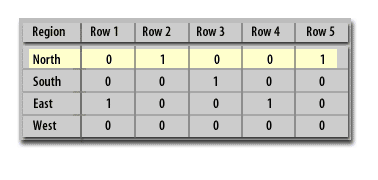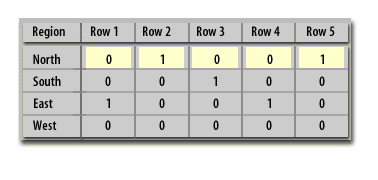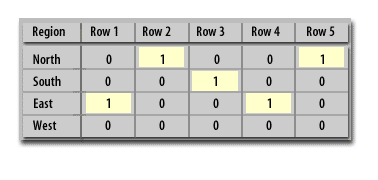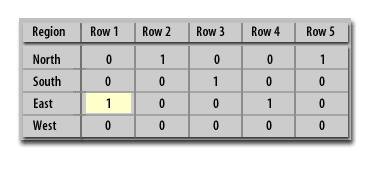Index Techniques   «Prev  Next»
 Lesson 2 Bitmapped Indexes Objective Describe B-tree and Bitmapped Indexes.

# Describe B-tree and Bitmapped Indexes

For high cardinality data or data with many possible values, B-tree indexes are most effective. B-tree indexes are most effective for high cardinality data, or data with many possible values. A common problem with traditional B-tree indexes occurs when an indexed column has low cardinality, which means it has too few distinct values to speed query access. For example, an index of a REGION column that has only 4 values (North, South, East, West) does not have enough distinct values to speed access for queries. It is an index column with relatively few distinct values, compared to the number of rows in the table, and is referred to as a low cardinality column. Oracle's answer to the problem of low cardinality is the bitmapped index.

## B-tree Indexes in Oracle

Traditional B-tree indexes are tree shaped indexes with individual index nodes to contain the high-level symbolic keys and pointer values. The b-tree indexing method refers to a balanced tree whereby tree nodes will split to create new nodes and spawn to create a new tree level as keys are added and deleted.

## Understanding B-tree Indexes

Problem: You want to create an index. You understand that the default type of index in Oracle is the B-tree, but you do not quite understand how an index is physically implemented. You want to fully comprehend the B-tree index internals so as to make intelligent performance decisions when building database applications.

Solution
An example with a good diagram will help illustrate the mechanics of a B-tree index. Even if you have been working with B-tree indexes for quite some time, a good example may illuminate technical aspects of using an index. To get started, suppose you have a table created as follows:

```create table cust (cust_id number,
last_name varchar2(30),
first_name varchar2(30));
```

You determine that several SQL queries will frequently use LAST_NAME in the WHERE clause. This prompts you to create an index:
```SQL> create index cust_idx1 on cust(last_name);
```

Note:
There is not an explicit CREATE INDEX privilege (although there is a CREATE ANY INDEX privilege). if you can create a table (which requires CREATE TABLE) then you can create indexes on it. You also need space quotas for consuming space in the tablespace the table/index is placed within. Keep in mind that with the deferred segment feature (available only with enterprise edition of database), that it's possible to create a table and index in a tablespace, but not realize the space quotas are required until a record is inserted into the table (and attempts to consume space).

Next several thousand rows are now inserted into the table (not all of the rows are shown here):
```insert into cust values(7, 'ACER','SCOTT');
insert into cust values(5, 'STARK','JIM');
insert into cust values(3, 'GREY','BOB');
.....
insert into cust values(274, 'ACER','SID');
```

After the rows are inserted, we ensure that the table statistics are up to date so as to provide the query optimizer sufficient information to make good choices on how to retrieve the data:
```SQL> exec dbms_stats.gather_table_stats
```
oracle strongly recommends that you do not use the ANALYZE statement (with the COMPUTE and ESTIMATE clauses) to collect statistics. oracle does support using the ANALYZE statement for non-statistics gathering uses such as validating objects and listing chained/migrated rows. As rows are inserted into the table, Oracle will allocate extents that consist of physical database blocks. Oracle will also allocate blocks for the index. For each record inserted into the table, Oracle will also create an entry in the index that consists of the ROWID and column value (the value in LAST_NAME in this example). The ROWID for each index entry points to the datafile and block that the table column value is stored in.

## Bitmapped indexes

We know that the purpose of an index is to provide pointers to the rows in a table that contain a given key value. In a bitmap index, a bitmap for each key value is used instead of a list of ROWIDs. Each bit in the bitmap corresponds to a possible ROWID. If the bit is set, the row with the matching ROWID includes the key value. A mapping function converts the bit position to an actual ROWID, so the bitmap index functions as a regular index although it has a different internal structure. If the number of different key values is small, bitmap indexes are very space-efficient.

## Region bitmap1) In a bitmapped index, a binary array is created with one index row for each table row2) Within each index row, there are entries for each index value (North, South, East, West)3) Across each index row, binary values are set to zero except for the index entry where the value is true. This value is set to 1.4) We can see that row 1 is in the East region
1. In a bitmapped index, a binary array is created with one index row for each table row
2. Within each index row, there are entries for each index value (North, South, East, West)
3. Across each index row, binary values are set to zero except for the index entry where the value is true. This value is set to 1.
4. We can see that row 1 is in the East region

Oracle Bitmap